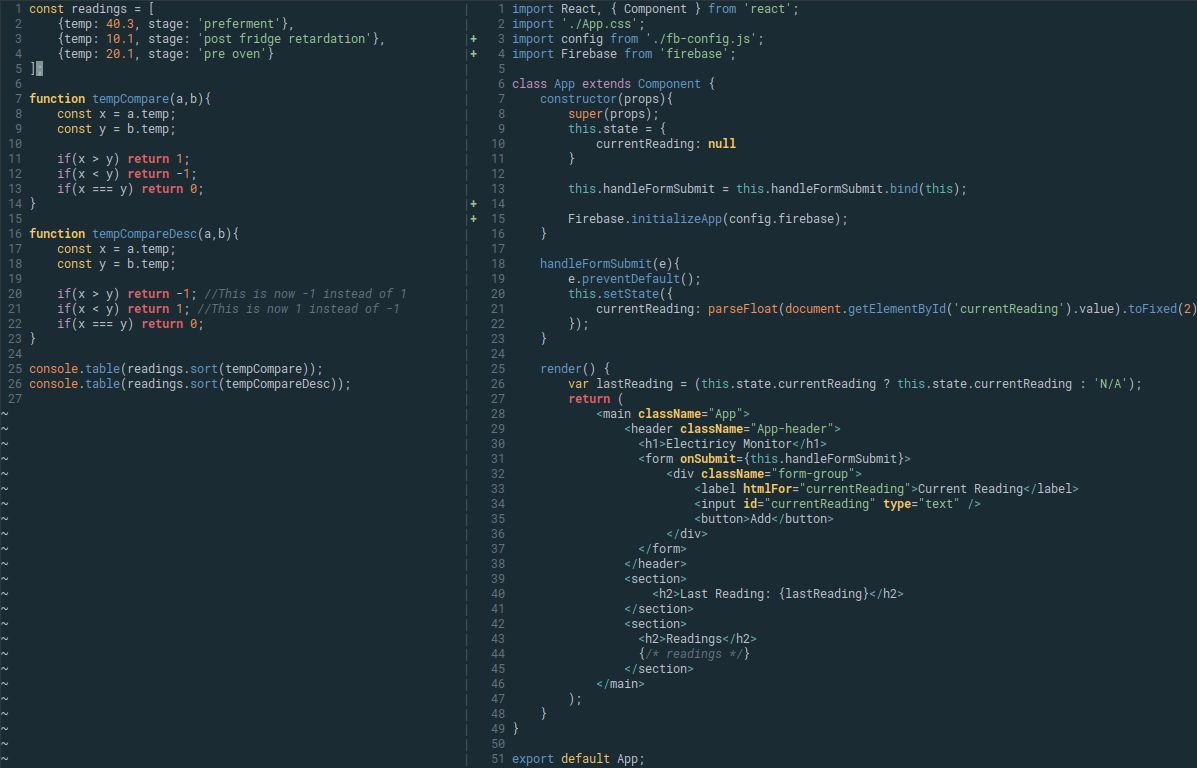// Huntly Cameron

# JS array sort with object keyIf you have a list of objects like so:

``````    const readings = [
{temp: 40.3, stage: 'preferment'},
{temp: 10.1, stage: 'post fridge retardation'},
{temp: 20.1, stage: 'pre oven'}
];``````

If you want to sort this list by `temp` and you try and run `readings.sort()` nothing will happen - it will return the array in same order as JS doesn't know what you're trying to do.  To help, you need to provide a custom compare function to sort.  A basic compare function to compare two numbers (a, and b) looks like this:

``````  function myCompare(a,b){
if(a > b) return 1;
if(a < b) return -1;
if(a === b) return 0;
}``````

All we need to do is tell this function to use our desired key:

``````  function tempCompare(a,b){
const x = a.temp;
const y = b.temp;

if(x > y) return 1;
if(x < y) return -1;
if(x === y) return 0;
}``````

Now we can run `readings.sort(tempCompare)` and you'll now have the following:

``````  [
{temp: 10.1, stage: "post fridge retardation"},
{temp: 20.1, stage: "pre oven"},
{temp: 40.3, stage: "preferment"}
];``````

If you wanted this list in descending order then you alter the return values in the `tempCompare(a,b)` function like so:

``````  function tempCompareDesc(a,b){
const x = a.temp;
const y = b.temp;

if(x > y) return -1; //This is now -1 instead of 1
if(x < y) return 1; //This is now 1 instead of -1
if(x === y) return 0;
}``````

Then you'll have:

``````  [
{temp: 40.3, stage: "preferment"},
{temp: 20.1, stage: "pre oven"},
{temp: 10.1, stage: "post fridge retardation"}
]``````